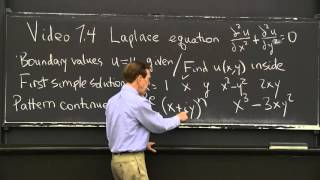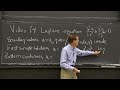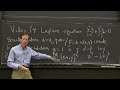# Laplace Equation

88K+ views   |   931 likes   |   20 dislikes   |
13:17   |   May 06, 2016

### Thumbs### Transcription

• Today I'm speaking about the first of the three great
• partial differential equations.
• So this one is called Laplace's equation, named after Laplace.
• And you see partial derivatives.
• So we have-- I don't have time.
• This equation is in steady state.
• I have x and y, I'm in the xy plane.
• And I have second derivatives in x and then y.
• So I'm looking for solutions to that equation.
• And of course I'm given some boundary values.
• So time is not here.
• The boundary values, the boundary
• is in the xy plane, maybe a circle.
• Think about a circle in the xy plane.
• And on the circle, I know the solution u.
• So the boundary values around the circle are given.
• And I have to find the temperature
• u inside the circle.
• So I know the temperature on the boundary.
• I let it settle down and I want to know the temperature inside.
• And the beauty is, it solves that basic partial differential
• equation.
• So let's find some solutions.
• They might not match the boundary values,
• but we can use them.
• So u equal constant certainly solves the equation.
• U equal x, the second derivatives will be 0.
• U equal y.
• Here is a better one, x squared minus y squared.
• So the second derivative in the x direction is 2.
• The second derivative in the y direction is minus 2.
• So I have 2, minus 2, it solves the equation.
• Or this one, the second derivative in x is 0.
• Second derivative in y is 0, those are simple solutions.
• But those are only a few solutions
• and we need an infinite sequence because we're going
• to match boundary conditions.
• So is there a pattern here?
• So this is degree 0, constant.
• These are degree 1, linear.
• These are degree 2, quadratic.
• So I hope for two cubic ones.
• And then I hope for two fourth degree ones.
• And that's the pattern, that's the pattern.
• Let me find-- let me spot the cubic ones.
• X cubed, if I start with x cubed, of course the second x
• derivative is probably 6x.
• So I need the second y derivative to be minus 6x.
• And I think minus 3xy squared does it.
• The second derivative in y is 2 times
• the minus 3x is minus 6x, cancels
• the 6x from the second derivative there, and it works.
• So that fits the pattern, but what is the pattern?
• Here it is.
• It's fantastic.
• I get these crazy polynomials from taking x plus iy
• to the different powers.
• Here to the first power, if n is 1, and I just have x plus iy
• and I take the real part, that's x.
• So I'll take the real part of this.
• The real part of this when n is 1, the real part is x.
• What about when n is 2?
• So we have x squared and we have i squared y squared,
• i squared being minus 1.
• So I have x squared and I have minus y squared.
• Look, the real part of this when n
• is 2, the real part of x plus iy squared,
• the real part is x squared minus y squared.
• And the imaginary part was the 2ixy.
• So the imaginary part that multiplies i is the 2xy.
• This is our pattern when n is 2.
• And when n is 3, I take x plus iy cubed, and that
• begins with x cubed like that.
• And then I think that the other real part
• would be a minus 3xy squared.
• I think you should check that.
• And then there will be an imaginary part.
• Well, I think I could figure out the imaginary part as I think.
• Maybe something like minus-- maybe it's
• 3yx squared minus y cubed, something like that.
• That would be the real part and that would be
• the imaginary part when n is 3.
• And wonderfully, wonderfully, it works
• for all powers, exponents n.
• So I have now sort of a pretty big family of solutions.
• A list, a double list, really, the real parts
• and the imaginary parts for every n.
• So I can use those to find the solution
• u, which I'm looking for, the temperature inside the circle.
• Now of course, I have a linear equation.
• So if I have several solutions, I can combine them
• and I still have a solution.
• X plus 7y will be a solution.
• Plus 11x squared minus y squared, no problem.
• Plus 56 times 2xy.
• Those are all solutions.
• So I'm going to find a solution, my final solution
• u will be a combination of this, this, this, this, this, this,
• this, and all the others for higher n.
• That's going to be my solution.
• And I will need that infinite family.
• See, partial differential equations,
• we move up to infinite family of solutions instead of just
• a couple of null solutions.
• So let me take an example.
• Let me take an example.
• We're taking the region to be a circle.
• So in that circle, I'm looking for the solution u of x and y.
• And actually in a circle, it's pretty natural
• to use polar coordinates.
• Instead of x and y inside a circle that's
• inconvenient in the xy plane, its equation
• involves x equals square root of 1 minus y squared or something,
• I'll switch to polar coordinates r and theta.
• Well, you might say you remember we had
• these nice family of solutions.
• Is it still good in polar coordinates?
• Well the fact is, it's even better.
• So the solution of u will be the real part
• and the imaginary part.
• Now what is x plus iy in r and theta?
• Well, we all know x is r Cos theta plus ir sine theta.
• And that's r times Cos theta plus i sine theta,
• the one unforgettable complex Euler's formula, e
• to the I theta.
• Now, I need its nth power.
• The nth power of this is wonderful.
• The real part and imaginary part of the nth power
• is r to the nth e to the in theta.
• That's my x plus iy to the nth.
• Much nicer in polar coordinates, because I
• can take the real part and the imaginary part right away.
• It's r to the nth Cos n theta and r to the nth sine n theta.
• These are my solutions, my long list of solutions,
• to Laplace's equation.
• And it's some combination of those,
• my final thing is going to be some combination of those,
• some combination.
• Maybe coefficients a sub n.
• I can use these and I can use these.
• So maybe b sub n r to the nth sine n theta.
• You may wonder what I'm doing, but what I'm achieved,
• it's done now, is to find the general solution
• of Laplace's equation.
• for an ordinary differential equation, a C1 and a C2,
• here I have these guys go from up to infinity.
• N goes up to infinity.
• So I have many solutions.
• And any combination working, so that's the general solution.
• That's the general solution.
• And I would have to match that-- now here's
• the final step and not simple, not always simple--
• I have to match this to the boundary conditions.
• That's what will tell me the constants, of course.
• As usual, c1 and c2 came from the matching the conditions.
• Now I don't have just c1 and c2, I have this infinite family
• of a's, infinite family of b's.
• And I have a lot more to match because on the boundary,
• here I have to match u0, which is given.
• So I might be given, suppose I was
• given u0 equal to the temperature was equal 1
• on the top half.
• And on the bottom half, say the temperature is minus 1.
• That's a typical problem.
• I have a circular region.
• The top half is held at one temperature,
• the lower half is held at a different temperature.
• I reach equilibrium.
• Everybody knows that along that line,
• probably the temperature would be 0 by symmetry.
• But once the temperature there halfway up, not so easy,
• or anywhere in there.
• Well, the answer is u in the middle, u of r and theta
• inside is given by that formula.
• And again, the ANs and the BNs come
• by matching the-- getting the right answer on the boundary.
• Well, there's a big theory there how do I match these?
• That's called a Fourier series.
• That's called a Fourier series.
• So I'm finding the coefficients for a Fourier series, the A's
• and B's, that match a function around the boundary.
• And I could match any function, and Fourier series
• is another entirely separate video.
• We've done the job with Laplace's equation in a circle.
• We've reduced the problem to a Fourier series problem.
• We have found the general solution.
• And then to match it to a specific given boundary
• value, that's a Fourier series problem.
• So I'll have to put that off to the Fourier series video.
• Thank you.

### Description

MIT RES.18-009 Learn Differential Equations: Up Close with Gilbert Strang and Cleve Moler, Fall 2015
View the complete course: http://ocw.mit.edu/RES-18-009F15
Instructor: Gilbert Strang

Laplace's partial differential equation describes temperature distribution inside a circle or a square or any plane region.